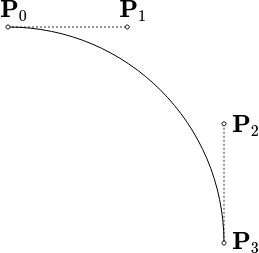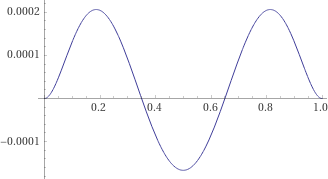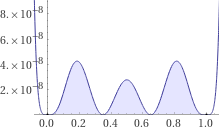# Least-squares approximation of the circle using cubic Bézier curves

We’ve already found a way to approximate a circle with cubic Bézier curves that has the smallest-possible maximum radial drift.

However, a doubt remains: If we allow the Bézier curve to drift slightly further from the circle—perhaps just momentarily—could we find an approximation with a better fit overall?

To answer this question, we’ll borrow a page from physics: The method of least squares finds the best fit while allowing for outliers by minimizing the sum of the squared residuals. We can apply the same method in continuous form to find the cubic Bézier curve that best fits the right circular arc.Figure 1: A cubic Bézier curve approximation to the right circular arc. We’d like to find the locations of the control points.

To do this, we’ll impose two constraints:

1. The endpoints of the cubic Bézier curve must coincide with the endpoints of the unit right circular arc, and their first derivatives must agree there.
2. The total squared radial drift must be as small as possible.

The general form of a cubic Bézier curve is:
,

The first constraint implies that:

This gives the parametric form of the cubic Bézier curve:

Our task is to find the value of which minimizes the total squared radial drift.

The radial drift is the radial distance from the cubic Bézier curve to the circle:

And the total squared radial drift is:

David Ellsworth found the optimal value of :

This is the least-squares approximation to the right circular arc:Figure 4: Radial drift in the least-squares approximation.

The least-squares approximation is almost the same as the earlier min-max approximation, but the maxima are slightly increased, and the minimum is slightly reduced.

You can see this in the graph of the squared radial drift:Figure 6: Squared radial drift in the least-squares approximation.

These adjustments slightly improve the total squared radial drift, but worsen the maximum radial drift. The total squared radial drift is in the least-squares approximation, which is better than the min-max approximation. The maximum radial drift is , which is worse.